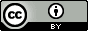Saturday, April 6, 2013

Fragment size and computational efficiency in fragmentation methods

I recently reviewed this interesting paper in which the FMO method is extended to four-body interactions (FMO4) and the fragmentation-size is decreased to roughly half a residue per monomer.  The latter was mainly done for analysis purposes, but some interesting results were also obtained for timings and accuracy.

For example, at the MP2/6-31G level of theory and using an FMO2 calculation using one residue per monomer as a reference, the accuracy and CPU cost is comparable to an FMO3 calculation if the fragment size is roughly halved.  The CPU increases only by a factor of 3 on going to FMO4, while the accuracy is increased by an order of magnitude.

In this post I explore this issue further using an idealized EFMO model. I write EFMO instead of FMO so I can ignore the macro-iterations associated the monomer energy, but I don't think will affect the conclusions much.

The total time has contributions from monomer, dimer, trimer, and tetramer ab initio calculations with all other terms being negligible.

$t_{EFMO4}=t_1+t_2+t_3+t_4$

The time for an ab initio calculation scales non-linearly ($\alpha >1$;$\alpha$ and all other Greek symbols are constant) with system size and assuming uniform monomer size so that $s_i=is$ ($s_1=s$):

$t_i=N_i s_i^\alpha=N_i i^{\alpha}s^\alpha$

If only dimers, trimers and tetramers contructed from nearest neighbor monomers need to be evaluated ab initio then the number of multimer $i$ is a linear function.

$N_i=\beta_i N-\gamma_i$

For a given system the monomer size and number of monomers is related by a constant

$sN=\delta$

i.e. a 10 residue protein can be constructed from five two-residue monomers or ten one-residue monomers.  Under these assumptions the CPU time is seen to increase non-linearly with monomer size. $$N_i=\frac{\beta_i\delta}{s} -\gamma_i$$ $$t_i\approx \beta_i\delta i^{\alpha}s^{(\alpha-1)}$$ So decreasing the monomer size will decrease the required CPU time.

Assuming a linear model $(N_i=N-i+1)$ and cubic scaling $(\alpha=3)$ and $sN=200$, e.g. a 200 residue protein described by 100 two-residue monomers ($N=100$), then the CPU time increases by a factor of four on going from EFMO2 to EFMO3$$\frac{t_{EFMO3,N=100}}{t_{EFMO2,N=100}}=3.9$$However, if the EFMO3 calculation is done with 200 monomers with half the size then the cost is not increased. $$\frac{t_{EFMO3,N=200}}{t_{EFMO2,N=100}}=1.0$$while the cost increases roughly three-fold on going to EFMO4: $$\frac{t_{EFMO3,N=200}}{t_{EFMO2,N=100}}=2.7$$Decreasing the size by a further factor of two $(N=400)$ one could expect a modest speedup  on going  to EFMO4$$\frac{t_{EFMO4,N=300}}{t_{EFMO2,N=100}}=0.7$$Going to a 3D example, for example a water cluster, where each monomer has a maximum of four nearest neighbors:$$N_2=2N$$ $$N_3=6N$$ $$N_4=10N$$ (I am not really sure about the last factor), then $$\frac{t_{EFMO3,N=100}}{t_{EFMO2_N=100}}=10.5$$ $$\frac{t_{EFMO3,N=200}}{t_{EFMO2,N=100}}=2.6$$ $$\frac{t_{EFMO4,N=200}}{t_{EFMO2,N=100}}=24.1$$ $$\frac{t_{EFMO4,N=400}}{t_{EFMO2,N=100}}=3.0$$ Increasing the scaling to quadratic $(\alpha=4)$ has relatively little effect on the relative scaling: $$\frac{t_{EFMO3,N=100}}{t_{EFMO2_N=100}}=15.7$$ $$\frac{t_{EFMO3,N=200}}{t_{EFMO2,N=100}}=2.0$$$$\frac{t_{EFMO4,N=200}}{t_{EFMO2,N=100}}=11.7$$$$\frac{t_{EFMO4,N=400}}{t_{EFMO2,N=100}}=1.5$$ The relative timings predicted by the 1D model agree quite well with the findings in the paper.  The 3D model is for an infinite system (i.e. no edge effects) and will overestimate the number of trimers and tetramers for a finite system.  The relative timings quotes for the paper was for 50 monomers.

The MAPLE worksheet I used for the calculations can be found here, and a pdf version here